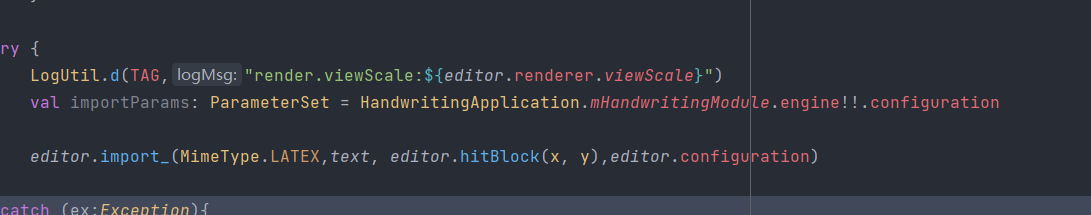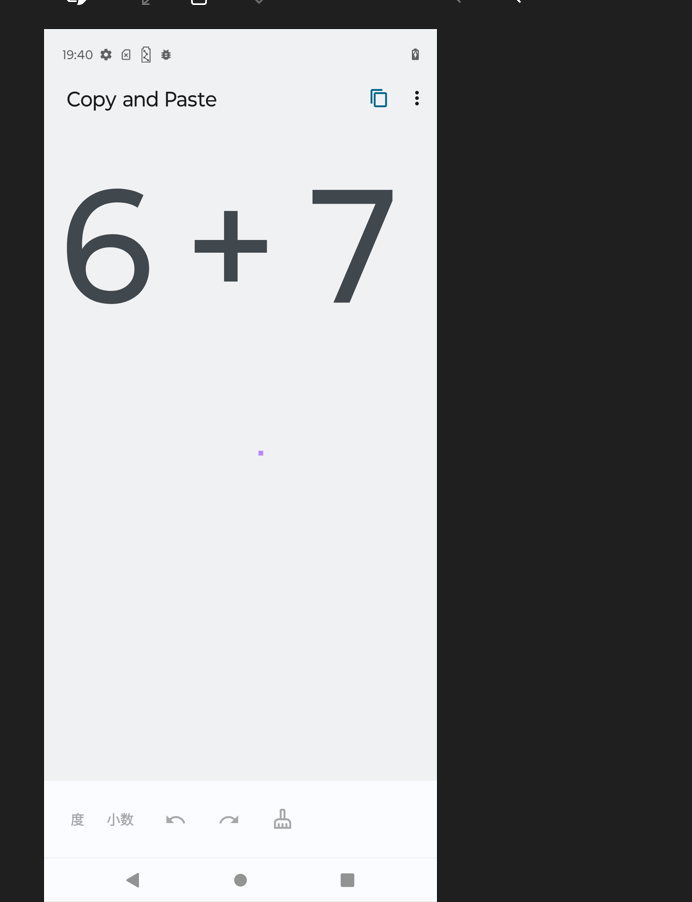# Forums

Math

## cannot get the theme when import the latex formula

when i import a latex formula, I can't paste it to the place where I press my finger, and the theme seems to be wrong. second  pic is in copy status ,third pic is in paste statusDear Heyb6,

thank you for contacting us.

Currently, This is as designed, by default when importing Latex content, it is not possible to set it to a given position, it will be placed at the top left corner.

If you want to add a math content at a give position, the only possibility is to import as JIIX (the latter contains the coordinates).

Best regards,

Olivier

Hi,Olivier,

In fact, I want to use jiix, but jiix does not meet our need to copy only one formula, when there are multiple formulas on the page. i don't know how to handle the bounding-box coordinates .

I alse have a few questions to ask.

1. How do we convert x and y coordinates into render coordinates in myscript? i know the way to  converts render coordinates into view coordinates (editor. render. transform)

2. There is a need to copy a single formula or paste the result to the view of the existing formula. Do you have a good idea. At present, I can copy a single formula, but I can't import it to the current view  without overwriting the existing content。

Best regards,

yibin

Dear Heyb6,

Thank you for the update.

In fact, I want to use jiix, but jiix does not meet our need to copy only one formula, when there are multiple formulas on the page. i don't know how to handle the bounding-box coordinates
>>Is it possible to have more information? For example, do you mean you have 3 equations, and only want to get one?
Normally, your JIIX file will contain several "expressions" (in theory, one per equation). Getting the expression you want to keep, you shall normally be able to re-create a JIIX file?
Then, after clearing the editor, you shall be able to import it as JIIX.

1. How do we convert x and y coordinates into render coordinates in myscript? i know the way to converts render coordinates into view coordinates (editor. render. transform)
>>If I understand well, you want to export the coordinates from the JIIX file, and add these again via the incremental API ?
Is there a reason for this?
Indeed, the coordinates in the JIIX are in milimeters. In order to convert these as pixels, you shall proceed as follows:
Transform tr= editor->getRenderer()->getViewTransform();
Point myPoint(x,y); //coordinates in milimeters
tr.apply(myPoint); //coordinates in pixels

=>You can then add them again to the editor

2. There is a need to copy a single formula or paste the result to the view of the existing formula. Do you have a good idea. At present, I can copy a single formula, but I can't import it to the current view without overwriting the existing content?
>>For a math part, we do not have any solution than clearing the editor before import. It is not possible to import into an existing content.

Best regards,

Olivier